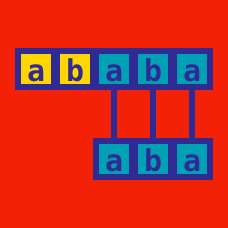Computer Science

# Shunting Yard Algorithm

$(8+3)\times (5-4)$

To evaluate the above expression, we use first convert it to reverse polish format using a shunting yard algorithm. Which of the following represent the correct order of reverse polish notation, when the above expression is evaluated?

$A) 8, 3, +, 5, 4, -, \times$

$B) 8, +, 3, \times, 5, -, 4$

$C) 8, +, 3, 5, 4, -,\times$

$D) 8, +, 3, 5, -, 4, \times$

$1\times 2 + 2 \times 5 / 7$

To evaluate the above expression, we use first convert it to reverse polish format using a shunting yard algorithm. Which of the following represent the correct order of reverse polish notation, when the above expression is evaluated?

$A) 1, 2, 2, 5, \times, /, 7, \times +$

$B) 1, 2, 2, 5, \times, \times, 7, / +$

$C)1, 2, \times, 2, 5, \times, 7, /, +$

$D) 1, 2, \times, 2, 5, /, 7, \times, +$

A polish expression or postfix expression is a notational form for logic, arithmetic, and algebra which places operators to the left of their operands. What is the value of the polish expression below when evaluated?

$\large{35\ 17\ 40\ 9\ - * + 7 - }$

×